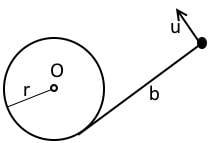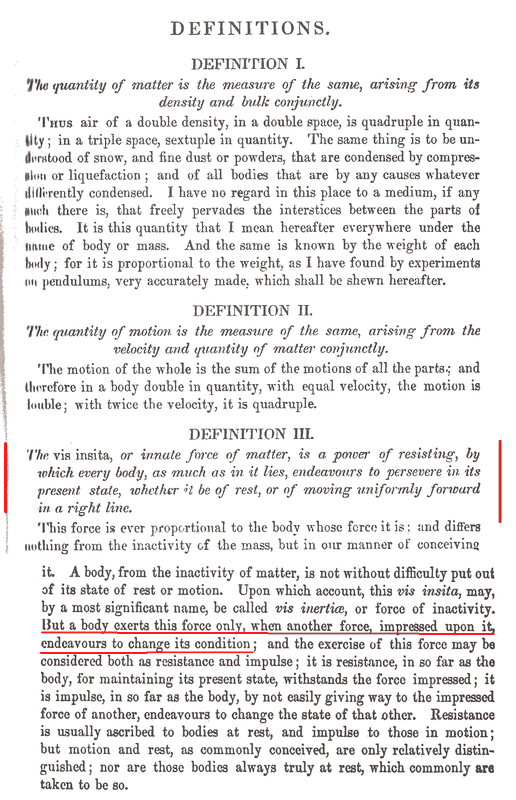# Rotation of a rigid body

haruspex
Science Advisor
Homework Helper
Gold Member
2020 Award
1. The disk rotates about its centre

2. The disk rotates about the point opposite to where the rope leaves the disk.

I prefer this argument. It is similar to the wheel on a car which, when viewed from a frame attached to the ground (as we have here), rotates about its point of contact with the ground. If there was friction then this is the intuitive answer suggesting that as I reduce friction to zero it will still rotate about that point.

3. The disk does not rotate because there is only one force acting on it.

4. The inertia of the disk provides "a restraint" which allows the single force to cause rotation. But, if so, how is the axis of rotation decided? Is it that about which the moment of inertia is the least - ie the centre of the disk?
I assume here you are referring to the point that is stationary, i.e. the instantaneous centre of rotation.
It is hard to guess this in advance. As I posted, it is best to consider the disc's motion as the sum of a linear acceleration of the mass centre and an angular acceleration about that centre.
If the tension in the string is T then, that being the only force acting horizontally on the disc, the linear acceleration is a=T/M.
The torque the string exerts about the disc's centre is RT, so the angular acceleration is given by ##I\alpha=RT##. With ##I=\frac 12 MR^2##, we have ##M\alpha R=2T##.
We can get the corresponding velocities at time t by multiplying each by t.
Suppose the instantaneous centre of rotation is distance X from the disc's centre, distance X+R from where the string leaves the disc. We have ##X\alpha=a##, so ##2TX=MX\alpha R=MaR=TR##, whence X=R/2.

Frodo
Gold Member
Frodo said:
shared it with another engineer, a maths graduate and a physics teacher and all were as confused as me.
We are electronic and electrical engineers respectively so mechanics was not a large part of the syllabus.
that is strange this is a very simple problem, not of high school level as I understand, but very simple
The only methodology we have been taught is (it was 50 years ago!)
1. work out what is happening and how it moves by examination
2. once you know what happens calculate the values.

We have never been taught the approach of setting up the equations and calculating the motion; nor Lagrangians, Hamiltonians or the principle of least action. I am now reading Equations of motion and, in a separate project, looking at least action as it is used in quantum theory.

wrobel
Science Advisor
Sorry for offtop. Perhaps it would be of some interest. I remember such a problem. On a smooth horizontal table there is a homogeneous disk of radius ##r## and mass ##M##. The disk is pivoted by its center ##O## and can rotate freely about the point ##O##. A weightless string is wrapped around the disk such that initially the tail of the string has a length ##b##. A particle of mass ##m## is attached to the end of the tail. Initially all the system is at rest and suddenly the mass ##m## is pushed with initial velocity ##\boldsymbol u## perpendicular to the tail.
Question: will the particle reach the disk?Frodo
Gold Member
Thank you. I will have a go at that by applying the equation(s).

haruspex
Science Advisor
Homework Helper
Gold Member
2020 Award
1. work out what is happening and how it moves by examination
2. once you know what happens calculate the values.
That's reasonable, but I would change it to "how it might move", and keep an open mind about the possibilities. As I showed in post #26, you cannot determine the instantaneous centre of rotation by inspection.

Frodo
Gold Member
The more I read about Newton and what he did the more impressed I am. He had the critical insight on this problem as Definition III in Principia.III in Principia.

•Lnewqban